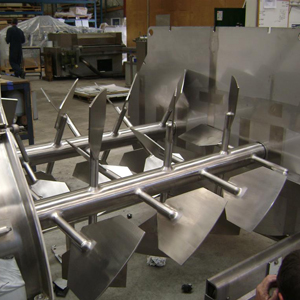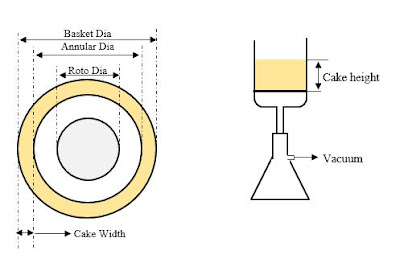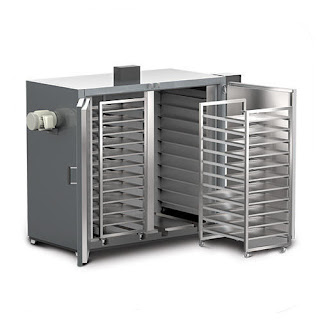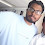# Pharma Engineering

For Engineer By Engineer

• ## Sunday, 10 February 2019

Hope everyone and everything is fine.
Today i gonna spend some time here posting about scale-up aspects that we may go through in our every day role role. I thought of posting this today because i've seen many of the engineers were getting slightly confused with that.

And here today i'll share the scale-up for three equipment's, i.e., Reactors, filters and drier.

Because these are most commonly used in pharma and based on receiving request/requirement i'll add other equipment's also.
Generally many of you think that scale-up is a difficult task, but as per me it is such a simple task and i'm considering it as a simple task because i'm concerned about only equipment here, not about the process and when we need to scale-up full pledge then even the process also need to mould based on requirement to meet the desired destination.

And i don't wanna waste time, so i'll be directly going into the matter and all the terms which will be new shall be defined in the flow itself.

[How to] Calculate moisture adsorbents required for packing?
[How To] Evaluate RC1e study report ?

Reactors Scale-up:

Scaling up for reactors is quite easy and if we know all the properties of them at a glance.
Basically for scaling of reactors we use 5 ways.

Already i've mentioned in one of my posts about these and there i've given it straight forward with constants, here i will make you explain them in detail.

The five ways are by maintaining constant,
1. Shaft speed,
2. Tip speed,
3. Reynolds number,
4. Froude number,
5. Power per unit volume.

And out of these all its quite difficult to scale-up based on power per unit volume, you may laugh at me but that's true and trust me it will produce much accurate results.

First of all we need to know the physical properties of reactor.
a. Length of reactor ( L ),
b. Internal dia of reactor ( D ),
c. Dia of the agitator ( d ).

We should calculate the
i.  Length ( L ) to Dia ( D ) ratio i.e., L / D and
ii. Sweep ratio i.e., Agitator Dia ( d ) to reactor internal Dia ( D ) i.e., d / D.

Case - ( A ): If both L / D and d / D of both reactors (Small scale & Large scale) are equal then we can proceed with Shaft Speed as both reactors can be said as Geometrically similar.

As in both cases with same RPM we can generate the similar mixing pattern as the skin friction will be same.

Whats is Skin Friction ?
During agitation the reaction mass will come in contact with reactor walls, at that point the reactor walls will provide some resistance and repel back the reaction mass into the center, the resistance shown by the reactor wall is known as Skin friction.

So, whatever the reactor RPM that we are maintaining in the small scale that can be blindly adopted to large scale without any calculation.  ------------- ( 1 )

Case - ( B ): If L / D is similar and d / D is not similar then we need to proceed further by scaling up based on the Tip Speed.

Tip Speed = 𝚷 x d x N,
Where N is RPM (Rotations per minute).

Let me explain you with an example,
Lets suppose we want to scale up from a Reactor - 1 which is having a Length of 2 m, Diameter of 1.5 m and agitator dia of 1.2 m. We need to scale upto a Reactor - 2 having a Length of 2.5, Diameter of 1.88 m and agitator dia of 1.5 m.

Now lets calculate the ratio's of two reactors,
Reactor - 1: L / D = 2 / 1.5 = 1.33, d / D = 1.2 / 1.5 = 0.8,
Reactor - 2: L / D = 2.5 / 1.88 = 1.33, d/D = 1.5 / 1.88 = 0.8.

As the both reactors having geometric similarity we can end our calculation with Shaft speed
So if Reactor - 1 is operated with 48 RPM, then simply adopt 48 RPm to Reactor - 2 also.

Lets suppose the Reactor - 2 is having a agitator dia of 1.3 m, then the ratio's will be like,
Reactor - 1: L / D = 2 / 1.5 = 1.33, d / D = 1.2 / 1.5 = 0.8,
Reactor - 2: L / D = 2.5 / 1.88 = 1.33, d/D = 1.3 / 1.88 = 0.69.

Here in this case, L / D of both reactors are same but d / D is not same, so we should go with tip speed.

So to meet the scale-up criteria we need to adjust the RPM, the RPM shall be calculated as,

( 𝚷 x d x N )reactor-1 = ( 𝚷 x d x N )reactor-2

N reactor-2   = ( d x N )reactor-1  / ( d )reactor-2

Let the RPM of Reactor-1 be 48, So the required RPM shall be

reactor-2  =  1.2 x 48 / 1.3 = 44.31 ~45 RPM.

This is what we call as Tip Speed ------------- ( 2 ).

Case - ( C ): If both L / D and d / D are not similar, then we need to proceed with Power per unit volume which is by maintaining a complete dynamical approach.

Simply, we can say that we are trying to equate the Power per unit Volume. i.e., whatever the power delivered by an agitator in small scale shall be matched with large scale.

Power per unit Volume ( P / V ) = Np x 𝛒 x ( N^3) x ( d^5 ) / V.
where,
Np - Power number,
𝛒    - Density,
V    - Volume of the reaction mass.

[How to] Select a condenser for a reactor?
[How To] Design a scrubber ?

Density of the reaction mass can be known, it wont be any issue,
Volume of the reaction mass can be known, it also wont be any issue, but coming to Power number, its quite complicated.

But many of the engineers will consider it as they know something based on their experience just like for Anchor power number will be 1.2, for propeller its 0.6, for PBT its 1.5 etc etc.

And if someone asks me the power number i will mention it as 50 or 60, because power number is not fixed and its an variable. Let me explain you we need to get the power numbers based on the agitator curves by plotting the Reynolds number.

Hence, for getting the Power number ( Np ), we need to calculate the Reynolds number( R ) first.

Reynolds number ( R ) = N x ( d^2 ) x 𝛒 / 𝛍.

Where,
𝛍 - Viscosity of reaction mass.

Now, let me show you the Agitator Curves of different agitators,Fig - 1: Agitator CurvesFig - 2: Agitator CurvesFig - 3: Agitator CurvesFig - 4: Agitator Curves
You may get a doubt, how may we generate Power number for a laboratory vessels, for those find below:Fig - 5: Agitator Curves

So, here we might get another doubt, that is for scaling up successfully through power per unit volume we will change the RPM of the agitated vessel, but for calculating the Reynolds number also we need the RPM.

[How to] Select a batch size during scale-up?
Guidelines for process development, Optimization

So i can tell that Reynolds number is impact the power per unit volume calculation indirectly, hence its appropriate to move further by scaling up based on equating the Reynolds number --------------- ( 3 ).

( Reynolds Number ) reactor-1  = ( Reynolds Number ) reactor-2.

If you want to proceed by scaling up with Power per unit volume, then we need to proceed with iterations, i.e., first of all we need to proceed with calculation which involves an assumed RPM which will be able to generate similar Reynolds number and Power per unit volume. ----------------- ( 5 ).

Finally there is another factor i.e., Froude's number, but this is only applicable for blending operations, especially for Powder processing. ----------- ( 4 )

Froude's Number = T / ( g x d )^0.5,

where,
T  - Tip speed,
g  - 9.81 (Constant),
d  - Agitator dia.

I can provide you additional information regarding these kind of mixing,

If Froude number less than 1 (F < 1 ), then the recommended agitators are Ribbon agitators.Helical Ribbon agitator

If Froude number is between 1 and 5, then the recommended agitator is twin paddle agitator.Twin Paddle agitators

If the Froude number is baove 5, then Shear mixers are recommended which will have around 90 - 95% of sweep dia w.r.t. vessel dia.Shear Mixers

That's it.........!!! Hope i've explained you in brief. If any queries pl contact me.

[How to] Reduce drying time in ANFD?
[How To] Select a filter for an oepration ?

Filtration Scale-up:

Now Lets go into Filters section.

Here i want to deliver about product filtration equipment's, especially about ANF's, PNF's and centrifuge. For detailed filters, visit this link Filters and their Significance.

Filtration Scale-up from Lab to Plant scale:

Basically in lab scale, filtration shall be performed in buckner filters by placing a watt-man paper or a filter cloth.

So for scaling up from lab to plant, the cake height that is observed in the lab buckner filter shall be considered in plant scale for successful scale-up and if the scale is need to be increased in plant it should be at calculated risk.

Let me explain you on calculation base, Let the observe cake height in Lab be 7 cms and the material bulk density be 0.8 Kg/L. In plant the available ANFD capacity be 0.28 Sq.m.

Lets start our show, the diameter of the ANFD is 0.6 m (obtained from ANFD chart).

Below is the ANFD size chart:

else we can calculate the dia based on cross sectional area formula.
Dia, d = 2 x [(0.28/3.141)^0.5] = 0.597 m.

The maximum volume of wet material we may scale-up is 0.28 x (7/100)
= 0.0196 m3 = 19.6 L.
So the wet material is 19.6 x 0.8 = 15.68 Kgs.

Hence based on the wet material, we need to reverse calculate batch size and fix it.

But we might know that its quite depressive that we are going to use a 0.28 Sq.m ANFD for getting 15.68 Kgs wet crude.

Hence, we should consider a buckner filter with lower dia, so the obtained cake height will be higher and will be helpful while scaling up to possible higher batch with available filter.

[How to] Perform material balance calculation ?
[How To] Calculate the vent size for pressure vessels ?

Lets suppose we have used a small sized buckner filter in lab and the observed cake height be 12 cms whereas in the previous case the cake height is 7 cms, now lets calculate the wet cake again,

Wet cake volume = 0.28 x (12/100) = 33.6 Lts.
Wet cake weight  = 33.6 x 0.8 = 26.88 Kgs.

So, here you can observe that the weight be 26.88 Kgs which is higher than the previous case i.e., 15.68 Kgs.

So, i can tell with proper data generation, the capacity can be increased with the available resources.

And a negative study i'll explain you, lets suppose you have generated data for a cake height of 15 cms in lab scale and the available ANFD capacity at plant of 0.125 m2 with a stroke height of 10 cms. Then it is not at all acceptable as if the stroke height is less than the cake height the expulsion of ML's would be difficult.

What is Stroke length ?
The length upto which the agitator of ANFD can raise to top from the filter screen is called Stroke length.

Now you can understand what i've previously delivered.

Below is the stroke length of different capacity ANFD:

If its a PNF, we can use same calculation but the cake height should be 75-80% of the lab cake height.

[How to] Map equipment's for a product manufacturing ?
[How To] Design a Chiller plant ?

Why its required to considered only 80% of the cake height observed in lab for a PNF ?
Because in ANFD there is a provision for agitation so that whenever during filtration cracks were observed on the material surface the observed cracks can be filled so that the applied pressure over the cake will be uniform, where as case of PNF, whenever cracks were observed channeling will happen and the pressure applied will escape and the uniform pressure will be missed.

Lab to Centrifuge:

If its the centrifuge we wan to perform the scale-up from Lab to plant, the just remember the cake height in lab shall be equaled to the annular cake radius of centrifuge.

Below is the pictorial representation of what i delivered above:Copyright Owner: Pharma Engineering

Scale-Up from Small batch size to higher batch size:

If its scaling up from small batch to higher batch size within plant, then its quite easy, and can be done by equating the cake height i.e., by maintaining same cake height in the higher batch size we can attain desired filtration rate and quality.

[How to] Design an agitator for an operation ?
[How To] Select a motor for an agitator ?

Drying Scale-up:

Usually in lab drying shall be performed in a mini tray drier by monitoring the chamber temperature.
But while extending the process to plant scale, there shall be many available drier i.e., ANFD, Cone driers, Tray driers etc

Drying in Tray drier:Fig: Lab tray drier

The total heat transfer area of the lab drier shall be calculated.
Let the dimensions of the lab tray be 4 x 6 cms with 3 trays,
Total surface area be 3 x ( 4 / 100 ) x ( 6 / 100 ) = 0.0072 Sq.m = 72 Sq.cm
Total material that need to be dried be 200 grams,
Then Scale-up factor for the tray drier is 200 / 72 = 2.78 grams/Sq.cm.

Now lets calculate the number of trays required in plant scale to meet the lab drying time.Fig: Plant Tray Drier
Lets suppose 50 Kgs of material need to be dried in tray drier, and each tray size of
20 x 32 cms. Each tray can contribute a surface area of 20 x 32 = 640 Sq.cm.

Total surface area required for drying = Total weight / scale-up factor
= 50*1000/2.78 = 17986 Sq.cm

Number of trays required = 17986 / 640 = 28.10 = ~29 trays.

So material shall be spread over 29 trays to match with the lab drying trend.

For a tray drier in plant scale, the scale-up factor shall be maintained and then the lab drying time can be successfully met in plant too.

[How to] Calculate energy of inlet steam ?
[How To] Select a motor for a pump & line sizings ?

Drying in ANFD:

Similarly we need to calculate the capacity of ANFD required for drying.

As per the Scale-up factor, the total heat transfer area required is 17986 Sq.cm = 1.79 Sq.m.
Considering 25% less than that of required will meet the requirement as the agitator shaft is provided with heating coil and along with the agitator it will contribute to the reduced 25%.

Considering 25% lower, total area required is 0.75 x 1.79 = 1.35 Sq.m.

Hence based on the scale-up factor to meet the requirement, a 1.5 Sq.m lateral surface area of ANFD is required.

Even if a larger capacity ANFD is preferred for drying, the minimum stirring height shall be considered, the cake height should be higher than that of the bottom agitator clearance.

Drying in RCVD:

For RCVD's the scale-up factor is not applicable. Drying can be done effectively, based on the selected occupancy.  For effective drying the occupancy of the wet cake in the RCVD shall be between 10-30 % and some times we might reach upto 50% and that will be like a calculated risk.

Lets suppose drying of wet material(50 Kgs) shall be done in a 500 L RCVD. Let the bulk density of the material be 0.4 Kg/L.
Wet cake volume is 50 / 0.4 = 125 L.

Occupancy = 125 / 500 = 0.25 x 100 = 25 %.

As the occupancy seems to be healthy, 500 L capacity of RCVD is efficient for drying the wet material.

That's it........!!! Hope i've made everything clear above for you.

Still any queries feel free to comment or message me ...........!!!

Related Posts:
[How to] Design a decanter for workups in Pharma ?
Agitated Thin Film Dryer Design calculations
[How to] Calculate vapour column diameter ?
[How to] Perform bond energy calculation ?

While performing the scale-up calculations, just remember "What consumes your mind, controls the calculation", So do it full-heartedly and carefully.

Above line is not from my own, but jus copied and slightly modified,
Credits to Chaitanya P(Process Engineer).

Hope everyone understand the above post, and you may got another doubt before going into the post  i..e, for a scaleup post why i've added a fish with bowls, the meaning it holds is in case of successful scale-up(jump) the fish will be safe or else the fish(quality) will die(Fail).Hi! I am Ajay Kumar Kalva, Currently serving as the CEO of this site, a tech geek by passion, and a chemical process engineer by profession, i'm interested in writing articles regarding technology, hacking and pharma technology.

1.Thanks for sharing good technical info
Regarding scale up in filtration,
In labs we use Buchner funnel in which constant pressure filtration technique is applicable,
If we plan a pnf or anf
Simply from the graph of t/V Vs V we can calculate alpha,Rm
With this we can calculate filtration time but
If we plan centrifuge in plant, filtration takes place on basis of centrifugal separation then
How to correlate the data from lab to plant

Regards,
Viswanath.

1.Dear Viswam,

What you said is correct, and above i've mentioned about only for maintaining the consistent purity and the alpha calculation can replicate the rate of filtration, as in lab there will be suction from bottom of buckner, so i've skipped the rate of filtration, however i'll try to extend the post.
As you asked in centrifuge there will be centrifugal force in action, hence the pressure applied in lab shall be equated to centrifuges RPM as like below,

Pressure = Centrifugal force / filtration Area = m x r x N^2 / A

m - mass of the wet cake, r is the radius of the basket, N is the rpm of the centrifuge,
A is the area of the perforated filtration area.

If any queries feel free to comment.

Best Regards,
AJAY K

2.Dear Aajay very good information

let me know, crystallization doing anchor agitator, now we are changing the agitator to propeller ok can we get same results after calculating the p/v valve

1.Dear Sir,

Its quite difficult to access the change, prior proceeding with the change its better to have FBRM(Focused Beam Reflectance Measurement) study in lab scale with change in agitator.

Regards,
AJAY K

3.How can i correlate the agitator

4.Good information Ajay

5.Dia, d = 2 x [(0.28/3.141)^0.5] = 0.597 m.......U have to consider A=Pi*r2...one side area of the circle of the cylinder is πr² is Used.....

1.Dear Nitin,

You are asking me to use A = pixr2, i've used A = (pi/4)xd2.

Both are from same mother.

Best Regards,
AJAY K

6.Can you please tell how to scale up the batch reactor where reaction as well as mass transfer is occuring

## This Blog is protected by DMCA.comHi! I am Ajay Kumar Kalva, owner of this site, a tech geek by passion, and a chemical process engineer by profession, i'm interested in writing articles regarding technology, hacking and pharma technology.